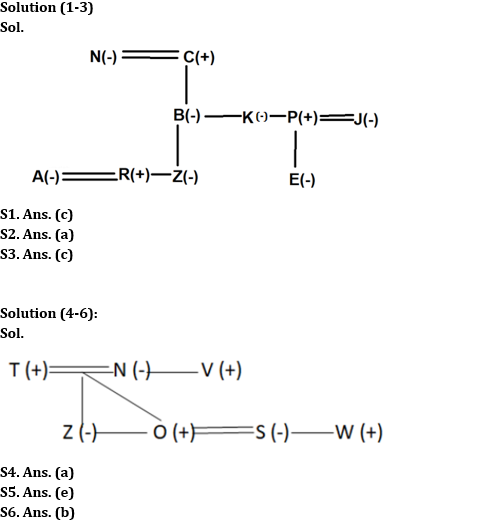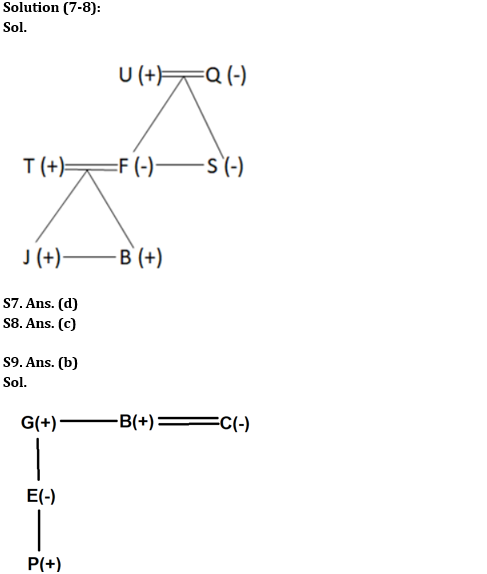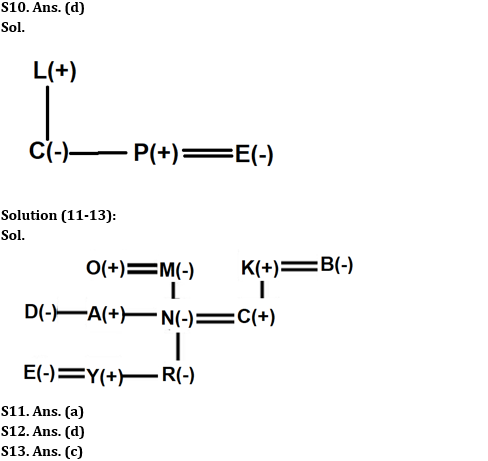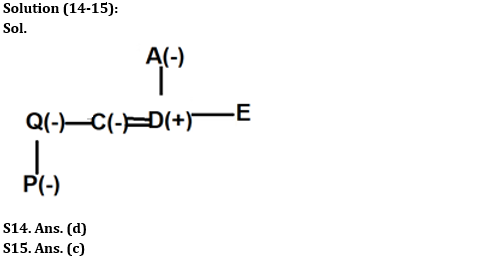Latest Banking jobs   »

# Reasoning Ability Quiz For IDBI AM/Executive 2022- 10th June

Directions (1-3): Read the following information carefully and answer the questions given below.
In a family of three-generation, there are ten members i.e. A, B, C, E, J, K, N, P, R, and Z, and three married couples. N is the wife of C. Z is the niece of P but not a child of K. A is the only daughter-in-law of E’s father’s sister. B is the daughter of C who is the father of K. N is the mother of P. Grandfather of E has only two daughters. K has no child. J is the wife of P who has only one child. E is the female member of the family but she is not the niece of P. No parent has more than three children.

Reasoning Quiz For IDBI AM/Executive 2022 10th June CHECK IN HINDI

Q1. What is the relation of P with respect to R?
(a) Either Uncle or Aunt
(b) Paternal Uncle
(c) Maternal Uncle
(d) Maternal Aunt
(e) None of these

Q2. How many female members are there in the family?
(a) Six
(b) Seven
(c) Either seven or eight
(d) Either six or seven
(e) None of these

Q3. Who among the following does not belong to the second generation of the family?
(a) Both K and P
(b) Both N and P
(c) Both E and R
(d) Both A and B
(e) None of these

Directions (4-6): Read the following information carefully and answer the questions given below.
Seven persons are there in a family with two generations. S is the sister-in-law of Z, who is unmarried female. T is the father of O and Z only. N is the sister of V and mother-in-law of S. W is the brother-in-law of O. The number of males is more than that of females.

Q4. How V is related to Z?
(a) Uncle
(b) Father
(c) Aunt
(d) Mother
(e) Can’t be determined

Q5. Four of the following five are alike in a certain way based on the given arrangement and so form a group. Who among the following does not belong to that group?
(a) T
(b) V
(c) O
(d) W
(e) S

Q6. How T is related to S?
(a) Uncle
(b) Father-in-law
(c) Father
(d) Mother-in-law
(e) Can’t be determined

Directions (7-8): Study the information carefully and answer the questions accordingly.
There are seven members in a family. The number of males is more than the number of females. T is married to F, who is the mother of two children. T has a sister-in-law. U is the grandfather of B and not the father of T. Q is the mother of S. J is the son of T who is the brother-in-law of S.

Q7. How T is related to B?
(a) Nephew
(b) Brother
(c) Uncle
(d) Father
(e) None of these

Q8. Four of the following five are alike in a certain way based on the given arrangement and so form a group. Which of the following does not belong to that group?
(a) F, U
(b) Q, B
(c) T, S
(d) J, U
(e) T, B

Directions (9-10): Read the following information carefully and answer the questions given below.
‘A%B’ means ‘A is the brother of B’
‘A \$ B’ means ‘A is the father of B’
‘A @ B’ means ‘A is the son of B’
‘A*B’ means ‘A is the husband of B’
‘A#B’ means ‘A is the daughter of B’
Now answer the following questions based on the above relations.

Q9. If the expression ‘P @ E#G%B*C’ holds true, then what is the relation of B with respect to E?
(a) Father-in-law
(b) Uncle
(c) Aunt
(d) Sister-in-law
(e) None of these

Q10. Which of the following expression shows that L is the father-in-law of E?
(a) L @ E%M#D
(b) M%L*B # E
(c) E \$ D @ L#A
(d) C#L \$ P*E
(e) None of these

Directions (11-13): Read the following information carefully and answer the questions given below.
In a family of three-generation, there are eleven members i.e. A, N, C, B, M, K, R, Y, E, O and D in which four married couples are there. A is the brother-in-law of C. Only one child of M is married. K is the paternal grandfather of R. N is the daughter-in-law of B. E is the married female member of the family. C has no siblings. M has only one son. Y is the nephew of D. Y is not child of A. D is the sibling of N. R is the granddaughter of M. O is the married member of the family and belongs to the first generation of the family. M is the mother in-law of C.

Q11. What is the relation of Y with respect to O?
(a) Grandson
(b) Son
(c) Uncle
(d) Father
(e) None of these

Q12. How many female members are there in the family?
(a) More than six
(b) Five
(c) Cannot be determined
(d) Six
(e) None of these

Q13. Who among the following is the paternal grandmother of R?
(a) O
(b) K
(c) B
(d) Cannot be determined
(e) None of these

Directions (14-15): Read the following information carefully and answer the questions given below.
‘A @ B’ means ‘A is the sister of B’.
‘A # B’ means ‘A is the son of B’.
‘A % B’ means ‘A is the daughter of B’.
‘A * B’ means ‘A is the mother of B’.
‘A \$ B’ means ‘A is the wife of B’.
Now answer the following questions based on the above relations.

Q14. If the expression ‘P % Q @ C \$ D # A *E’ holds true, then which of the following statement is definitely false?
(a) D is the husband of C
(b) Q is the sister-in-law of D
(c) P is the niece of C
(d) E is the uncle of C
(e) None of these

Q15. If the expression ‘P % Q @ C \$ D # A *E’ holds true, then what is the relation of A with respect to C?
(a) Son-in-law
(b) Daughter-in-law
(c) Mother-in-law
(d) Father-in-law
(e) None of these

Solutions#### Congratulations!